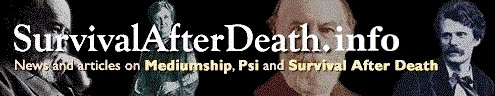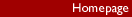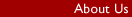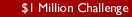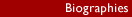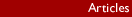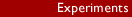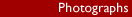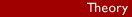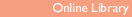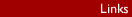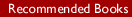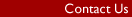ONLINE LIBRARY Book: "Intelligence Behind the Universe!" Author: Ronald D. Pearson B.Sc (Hons) Availability: From Michael Roll Contents / Previous Chapter / Next Chapter - Chapter 2 - Historical Background The Problem of Wave-Particle Duality ___________________________________________ The problem of wave-particle duality is rooted deep in history. Even during the lifetimes of Galileo and Newton conjecture arose concerning the nature of light. Did it propagate as a system of waves or did it consist of a stream of particles? Even today no solution has been established. The history is in itself extremely interesting and so this chapter concentrates on its development. At the same time all the essential knowledge of physics, needed for a total appreciation of the climax of our theme, should be imparted to any intelligent layperson. We cannot deal with just light alone because other factors are involved, such as some of the basic laws of physics. By presenting the subject matter as a brief historical account, however, it is hoped that a painless introduction to a fascinating subject will be provided. Then the reader will be able to appreciate the difficulties of interpretation facing physicists. The data seems crazy and conflicting. It will be shown that even today none of the solutions to the problems raised by wave-particle duality, which have been tentatively accepted by physicists, are really satisfactory. And everybody knows this, so the search continues. What is thought to be a new and novel solution is described in later chapters. The description is purely verbal - no mathematics is needed. Yet it is shown capable of satisfactorily relating known data to a remarkable degree. It is for the reader to judge its success. The solution started out following established lines with the assumption of an accidental origin of the universe in mind. This became more and more untenable as a solution emerged. Finally the solution described homes in on a creationist scenario which appears to justify the faiths people have relied upon throughout the ages. This also answers the oft-posed question, "How could God have made himself?" It shows that nature allows options supporting the idea that people could have souls. Physics cannot prove they have them but experience has shown that nature abhors a vacuum. All possible niches seem to be filled. 2.1 Reading Plans The reader having no technical or scientific background is likely to find some of the concepts introduced difficult to comprehend at the first reading. If this is the case then a more leisurely study is recommended. The difficulties being encountered probably arise from a too rapid introduction to one new concept after another; it takes time for the mind to accept them. None are really difficult though they may appear so at first. It is best to read a little and then come back to it later, giving the subconscious mind time to digest. The mind often seems to act like a separate organ, sometimes acting contrary to our wishes. It is necessary to learn how to trick it into doing what we want. If interest is found to wane a little then a quick look at bits of Chapters 9, or 13, followed by a rest period, should do the trick. These two chapters, however, only show how the new theory can explain psychic phenomena and spirituality in terms of the "Grid", a construct developed by the new theory. It is a computer-like structure pervading all matter and space to interconnect everything to everything else. It provides the invisible base on which matter is organised so that the illusion of a solid world is created. This is needed for finding a plausible solution to the dilemmas posed by wave-particle duality and also for resolving a contradiction in explanations for the shapes of atoms. For a brief view of this without too much detail, Chapter 8, which summarises the solution, should be found helpful and, perhaps, more readily digestible. Alternatively one or two of the very simple experiments described in this chapter can be tried. Each apparatus is quickly and easily constructed and set up. Science develops with experiment and theory advancing together hand in hand. Experiment helps new concepts to gel in the mind. For those who find they can relate without excessive effort to the logic of the scientific way of thinking, the best approach is to read straight through. This way should convey the deepest understanding of the new extension to physics that I am trying to communicate. 2.2 Galileo Galileo Galilei, according to Shamos(120), was born the same year as Shakespeare, in 1564, and is the recognised father of the scientific age. The ancient Greeks had worked in abstract ways to develop mathematics and were very successful in their efforts. They did not, however, attempt to use it together with practical experiment to provide a true science. This was the step that Galileo made. He rolled cannon balls down inclines to measure the rate at which their speeds increased. In other words he measured their "acceleration" and formulated theories that enabled the mechanics involved to be understood. He found, for example, that however massive the ball was, on a given incline the acceleration was always the same. Yet the heavier the ball the greater would be the force or distance needed to bring it to rest after it ran on to the level. To obtain a single quantity which would specify the effort needed to bring any ball to a stop he multiplied the mass, found by weighing, by the velocity and called the product "momentum". This is a very important property in all mechanics. Both the velocity and mass contributed to the effort required to stop any object. Any object showed a reluctance to change its motion. If at rest a force needed to be applied to start it moving; when in motion a force of some kind was required to stop it moving. In other words it possessed "inertia" and momentum provided a measure of this property. Momentum will come up again quite frequently as our story unfolds. He also experimented with pendulums. Indeed a surprising degree of insight into the mechanics governing our universe can be gleaned by studying the simple pendulum. He discovered that, provided the weight of the thread was negligible in comparison with the compact pendulum bob it supported, the time required to complete one swing was the same no matter how heavy or from what material the bob was made. The period was also unaffected by the angle of swing up to about a 30 degree inclination to the vertical. This so-called "periodic time" only depended on the distance between the centre of the bob and the point of suspension. To obtain a periodic time of one second, for example, this distance needs to be 24.8 centimetres. To treble this period the length needs to be nine times as great. In general the length required needs to be increased in proportion to the square of the periodic time specified. Then he tried placing round pegs below the suspension point so that a new pivot was created at the half-swing position. The second half-period was then greatly reduced as compared with the first and the angle of swing increased. The most interesting observation he made, however, was that when the bob rose to the point where momentarily it came to rest, it always reached the same height, above a level floor, as the height from which it was released. This may not at first seem very remarkable but, nevertheless, the implications are profound. The Interpretation accepted, even today, is that two different forms of energy are involved which interchange with one another. As the bob swings down its speed increases and associated with this speed is an energy of motion called "kinetic energy", which we will subsequently denote by the symbol "KE". Because this energy has to come from somewhere it is assumed to derive from reduction in height under the action of gravity. At a high level the bob will possess more so-called "gravitational potential energy" (GPE) than at a lower level. An arbitrary level can be chosen as the datum from which measurement is to be made. This could be conveniently chosen as the lowest point reached by the bob. Then at the highest point, where the speed falls to zero, all the kinetic energy will be recovered as an increment of GPE. When it swings back to the lowest point again all the increment of GPE stored will be converted back again to KE. With this explanation even though symmetry of the oscillation has been destroyed by adding a peg, the bob must rise by the same height increment in order to convert a given amount of KE to GPE. Furthermore an oscillation consists of repeated interchange between GPE and KE and could go on forever in principle. Galileo used pendulums as timing devices for his experiments but the amplitude of the swings always decayed to an eventual stop. This was caused by parasitic effects such as air resistance which absorbed a little of the energy at each swing. To offset this loss it was necessary to give the bob a little push at the start of each period, a push carefully timed to match the natural period. This is what the "clockwork" mechanism ultimately achieved. These experiments of Galileo are well worth trying and are easily performed. He made other important contributions we will not mention. Unfortunately his astronomical observations supported the theory of Copernicus (1473-1543). This was the "heliocentric theory", which displaced the Earth from its special position as the centre about which the Sun and indeed the whole universe was believed to revolve. This was perceived as a threat by the established Church because it ran counter to their teachings. As everyone knows, Galileo was forced to recant by the Inquisition. So conflict between science and religion arose right at the beginning of the age of science. Only now, late in the 20th century, does the rift promise to heal. Wave-particle duality, one of the two main topics of this book, is the key factor in this new turn of events, as will be shown. It arose when somebody else began studying the behaviour of light. A painter 54 years younger than Galileo worked for the Pope and must also have been a gifted person. He was Francesco Grimaldi (120). He experimented with light by allowing it to pass through small apertures and discovered some interesting effects. It is very easy and informative to try and reconstruct some of the tests he made. He used the Sun of course, but it is easier to use a slide projector. This can be set to focus in the far distance to obtain a parallel beam. Then pieces of thin cardboard can be used as masks to partially obstruct the beam, which is finally allowed to fall upon a white screen about two metres away. If a slit about 3mm wide (1/8") is made by a sharp knife, then most people would expect to see a thick bright band of light displayed on the screen. Instead, what is actually seen is a whole array of light and dark bands, together with some rainbow colours, covering the screen. Now a second Piece of card with a similar slit cut in it can be held vertical and a few inches behind the first. The card can be rotated so that the new slit is inclined at about 45 degrees to the first slit. Then a cross-hatched pattern covering an oval patch of light will appear. These tests are very interesting and are well worth trying. To Francesco this seemed analogous to the "interference patterns" which can arise on a pond. These occur when two pebbles are thrown in together. The Subsequent ripples mutually interfere in the region where they cross over one another. He deduced from this that light must have a wave nature. When one realises the state of knowledge at the time, this was a remarkable deduction. Later both Christian Huygens (1629-1695) and Robert Hooke (1635-1703) supported this conclusion. Unfortunately they were contemporaries of Sir Isaac Newton, who produced his "corpuscular theory" for light. He imagined light to propagate rather after the fashion of a stream of tiny machine-gun bullets. Each was a separate "corpuscle". Nowadays these are called "particles". 2.3 Newton's Physics Newton (1642-1727), probably the greatest genius of all time, is famous for creating a whole new system of physics which still forms the basis of most scientific and engineering calculations. He developed a new form of mathematics, his "calculus", and used it to explore his new ideas of mechanics. He defined what energy is and showed this to be quite different from the concept of momentum. He caused balls to bump into other balls and measured the speeds before and after collision. By interpreting the results of such experiments he made a startling discovery. A simple mathematical "law" existed from which the outcome could be predicted. This is called "The Law of Conservation of Momentum". He found that if he added up the momentum (mass times velocity remember) of two balls after collision this would always be equal to the sum of the momenta the balls had before collision. This applied if the balls were constrained to move only along a single line with one of them stationary before impact. It also applied if both balls were moving before impact in the same direction. If they moved in opposite directions before or after impact, then the law worked provided the velocity of one direction was regarded as negative with respect to the other. The direction of motion was as important as the speed. This is where the term "velocity" differs in its meaning from "speed". Speed and velocity are measured in the same units such as miles per hour or metres per second (m/s) but speed is called a "scalar" quantity because the direction of motion is not included. Velocity is a "vector" quantity, meaning that speed and direction are combined. There is a more general meaning to change of velocity which applies where motion is not constrained to a straight line. The difference in meaning between speed and velocity can then be provided, for example, by imagining the effects of cornering in a car at high speed. The car moves round the corner at a constant speed but the velocity is continually changing because of the changing direction. The rate of change of velocity precisely defines what is meant by "acceleration" according to Newton. He then showed that when a mass is accelerated a force is involved. When a car is accelerated forward, for example, the people inside are also accelerated and consequently feel a force pushing them as they press against the backs of their seats. Here, simply considering the speed increase alone is sufficient because direction is unchanging. But when cornering at constant speed a side force is encountered. Again this force is associated with an acceleration but this time it is due to the rate of change in direction. To make an object move in a circular path it is necessary to provide a force of constant magnitude but always directed at right angles to the direction of motion, then no change of speed arises. An example is the swinging of an object on a string to make it travel in a circle. The string applies the necessary force. Of course, in the general case, both the speed and direction can be changing simultaneously. Because of similar observations, the term "mass", which represents the amount of matter present in an object, was defined by Newton from the force required to Provide a given acceleration, or: "Mass is equal to the force applied divided by the acceleration produced". This is one of his laws. Newton also produced a theory of gravitation which said that a force was somehow transmitted through empty space between any pair of objects. This force tried to pull them together. One object could be the Sun the other a planet. The force was proportional to the mass of one multiplied by that of the other and divided by the square of the distance separating them. This is the well-known "inverse square law". It means, for example, that by trebling the separating distance the attractive force will be reduced to one ninth of its previous value. The objects would not fall into one another by the acceleration produced by this force if at the same time a sufficient "tangential" velocity existed. This is the velocity measured at right angles to the direction of force. In special cases the result could be perfectly circular orbits. It will be remembered that a circular path is traced by an object which is acted on by a constant force always at right angles to the direction of motion. Then Newton showed, by clever mathematical analysis, that in general the orbits of planets would be perfect ellipses. These are oval shapes with the Sun displaced from the centre. The point of greatest distance from the Sun is called the "apogee" and here the orbital speed is a minimum. At closest approach, the "perigee", the speed is a maximum. For the ellipse, acceleration is due to both change of speed as well as direction. For those who would like a little more insight about Newton's laws, an appendix is attached at the end of this chapter. It explains the differences in meaning between the concepts of kinetic energy and momentum and describes momentum conservation in two dimensions. It also describes another simple pendulum experiment the reader might like to try which demonstrates the conservation of momentum and energy. More important for our problem of wave-particle duality is that light, which according to Newton is made from particles, would behave no differently from other objects. A beam of light from a star grazing the "limb" of the Sun (its edge) would be deflected by the Sun's gravitational pull. The star would appear to have been deflected from its normal position in the sky. No such deflection would arise if light propagated only as a system of waves. So great was Newton's influence that his opinion was not officially questioned for more than a hundred years. But this was the start of the dilemma. Did light propagate as a system of waves or did it have a particle nature? 2.4 Young's Two-Slit Experiment Thomas Young (1773-1829) answered this question in favour of waves, though not until 1803. He is famous for his "two-slit experiment". He was a physician, mostly an eye specialist, but made contributions to a surprisingly large number of other disciplines such as Egyptology, science and engineering. "Young's modulus", for example, is a key factor for engineers which enables the deflections of structures to be calculated. His two-slit experiment showed light must have a wave nature, being propagated after the fashion of ripples on a pond, though in all three dimensions instead of the two which can specify the surface of a pond. He used a beam of light from the sun, reflected by a mirror, to pass horizontally through a small hole in a blind and into a darkened room. Then he allowed it to pass through a pair of narrow slits arranged vertically side by side and therefore parallel to each other. Further on, a screen was arranged to display the resulting pattern. If most other people at the time had tried this, they would have expected to see a pair of bright lines on the screen. But not Thomas Young. He saw what he was expecting. But this consisted of a pattern of light and dark stripes aligned in the same direction as the slits and extending sideways indefinitely as it faded away. This "interference pattern" is illustrated in FIG.1 and can only be explained by the superimposition of wave trains. Instead of forming fairly sharp shadows, as would be expected from the stream of particles concept, the light spread out by "diffraction" at the edges of the slits, forming two sources of waves in phase with one another. This means that if the waves are pictured as ripples, then the maximum heights are reached at equal distances from the Slits. At the centre of the screen both sets of waves arrive together so reinforcing one another to cause "constructive interference" and produce a bright band. These light and dark bands are only seen edge on in FIG.1, where the screen, shown at the top of the figure, intercepts the light.The waves arrive out of phase at points on the screen away from the centre and at a certain distance total "destructive interference" occurs. The crests of waves from one slit are neutralised by the arrival at the same instant of the troughs from the other. Hence a dark band is seen. Farther away still, the peaks of the nearest slit arrive a full wave cycle before those from the other and so peaks again coincide to produce another bright band or "fringe". The process repeats indefinitely, so that many alternating bright and dark fringes are formed. FIG.1 should be interpreted as an instantaneous frames in time, such as would be recorded on a cine film. All the waves shown are to be imagined as progressing up the page and spreading outward until they hit the screen. The places where the wave crests cross each other can be seen. Here the maximum wave amplitudes arise and to either side, for a substantial distance, a high value is maintained. These regions are marked by thick lines. The pattern can be easily reproduced by a ripple tank. A shallow tank containing water to a depth of about an inch can be used with the slit masks arranged in vertical planes fixed to the flat bottom. Slits about 0.1" wide and separated by about 1" will be found satisfactory. The ripples can be formed by making use of the vibrations of a spin drier. A strip of metal glued to the frame of the drier can be bent to dip into the water at a place representing the light source. It is important that the experimental tank has a mounting separated from the spin drier. The screen needs to be represented as a sloping beach to cause waves to break and so prevent reflection. Young's real experiment can be used to actually measure the "wavelength" of light. This is a measure of the distance between successive wave crests or, alternatively, their troughs. A colour is selected by a filter. This means that a certain wavelength has been selected. Then the distance to the screen, the separation of the slits and the pitch of the fringes are measured. By quite simple geometry the wavelength can then be calculated. It is simply the centre-to-centre distance between the bright fringes, multiplied by the separating distance of the slits and divided by the distance of slits to screen. It has been found in this way that red light has a wavelength of 7/10,000 of a millimetre whilst blue light is shorter at 4/10,000 mm. These values mark the boundaries of human vision. But beyond vision at longer wavelengths "heat rays" can be detected which are called "infra-red". "Ultra-violet" rays, also invisible, can be detected at wavelengths shorter than the blue. The heat radiated by a hot object can be felt. Then as the temperature is increased it is seen to glow dull red as well. Further heating causes the colour to change to yellow. A very hot object like a lightening flash is seen to be blue. By some strange quirk we, as people, relate blue to cold and red to hot. From the scientific viewpoint this is the wrong way round. Although Young backed his experiments with a convincing theory, not much impact was made at the time. But ten years later Jean Fresnel, who is famous for his lighthouse lenses, produced an even better theory, showing how diffraction could be explained. This definitely established the wave nature of light. Then Clerk Maxwell (1831-79) showed light to be electromagnetic in nature. He used abstract mathematical reasoning of a high order to produce "structural equations" but these were not physical structures able to be visualised. They did show, however, that light consisted of "transverse waves". This means a motion occurred at right angles to the direction of propagation, like that produced by the shaking of a skipping rope. They were a combination of electric and magnetic force fields moving in sympathy with one another. Light represented only a small part of the electromagnetic spectrum which extended beyond the infra-red to radio waves which could have wavelengths of many metres. Beyond the ultra-violet, radiation of very short wavelengths was discovered. These were the so-called X-rays, having great powers of penetration. All these waves moved at the same speed, that of light which is still represented by the symbol "c". This had been measured by a contemporary of Newton, the astronomer Olaus Roemer, who gave a very accurate value. This speed is 300,000 km/s (kilometres per second). A few simple equations will now be quoted. If the reader feels put off by this in any way then a brief look at the appendix may help. It must be emphasised at this point that this book will not quote many equations and if they are ignored it will not really matter. The frequency "v" at which waves arrived at a given point was simply connected to the wavelength "k" by dividing "C" by "k" i.e. "V = c/k. If, for example, "k"= 10m, then this equation shows the corresponding frequency to be 30 million cycles/s, which is usually stated as 30 megahertz. The frequency or, alternatively, the wavelength was all that differentiated light from other types of electromagnetic wave. Many other famous people such as George Stokes and H.A. Lorentz added their contributions in support and so there seemed absolutely no doubt that light had purely a wave nature. 2.5 Planck and Einstein Until Planck and Einstein arrived on the scene! The German physicist, Max Planck (1858-1947), had shown in the year 1900 that electromagnetic radiation had to be emitted in discrete packages of energy which could not be further sub-divided. These packages were called "quanta" and were larger the higher the frequency "V" of the radiation. The energy value corresponding with each quantum of radiation is given by "h.v", where, appropriately enough, "h" is known as "Planck's constant". It appears repeatedly throughout the whole of quantum physics. A beam of light would carry a total energy equal to the number of quanta transmitted multiplied by the energy carried by each. The rate of energy falling on every square metre of a surface defined the "intensity" of light and this would be increased by either increasing the number of quanta concentrated in the beam or by increasing their frequency. The puzzle is how to relate a frequency to particles. Later an answer will emerge. Then in 1905 Albert Einstein (1879-1955) investigated the "photoelectric" effect. He called each quantum of light a "photon". Each penetrated the surface of a metal and gave up all its energy to a single "electron". At this point it is necessary to give some idea of the way atoms are structured. 2.6 The Atom The ancient Greeks defined the atom as the smallest part into which matter could be subdivided. These were thought of as little hard balls. An element such as iron or sulphur can be divided to leave such isolated atoms, but although they seem in many ways to be solid and hard, what we sense is far from a true description of reality.In fact, as shown by the scattering experiments of Sir Ernest Rutherford (1871-1937), they consist of a nucleus occupying only a minute fraction of the total volume, yet accounting for nearly all the mass of the atom, surrounded by even smaller and lighter particles, the electrons. The latter were discovered by J.J. Thomson in 1897. They are credited with "negative electric charge" and possess a total which is balanced by an equal amount of positive charge in the nucleus. There is a force of attraction between negative and positive charge and it is this which textbooks say cause the electrons to be held in so-called "orbitals" close to the nucleus. It is the orbitals, the space in which the electrons are constrained to move, which defines the size of the atom. They appear hard because the orbitals of other atoms contain electrons also and these exert forces of repulsion upon one another. This prevents the orbitals of adjacent atoms from inter-penetrating to more than a very limited degree, even when pressed against one another with great force. There is a limiting force, then the atoms collapse to provide the very high densities of the "white dwarf star". When an ordinary star, like our familiar Sun, has burned its nuclear fuel, it first expands to a "red giant" and then by losing heat, contracts to a white dwarf. The atoms are crushed by immense gravitational force, now that the radiation pressure caused by light trying to escape is no longer present in sufficient amount to offset gravity. It now has the mass of the Sun compressed into a ball no bigger than the Earth. Yet the mass of the Sun is 333,400 times the Earth's mass! Even this does not represent the ultimate density of matter. Larger stars sometimes explode into supernovae and their centres are imploded to become neutron stars. Then one solar mass is contained in a ball only a few kilometres across. This density corresponds with that of the atomic nucleus. But even this is not the Ultimate density because the nucleons are themselves made up of tiny components occupying only a small fraction of the available space. Hence electrons are sub-atomic particles having unit "negative electric charge". This charge causes mutual repulsion with other electrons and a flow of such charges constitutes an electric current. They react by a force of attraction to any positive electric charge. Such charges are carried by the "protons" which form Part of the atomic nucleus. Electrons are arranged in cloud-like form defining the orbital about the relatively massive atomic nucleus. The electron clouds of adjacent atoms stick to one another by short-range residual electromagnetic forces. Magnetic forces result from electric charge in motion. The electrons have both a spinning and an orbital motion, so that magnetism is generated. Then other electrons respond to this magnetism as well as the electric force. In materials like iron the magnetic force builds up from atom to atom and then becomes very evident. The combination of electric and magnetic forces holds atoms together to make apparently solid structures or liquids. In FIG.2 a few atoms are illustrated forming a crystal having a cubic lattice. Electron clouds, confined within volumes of space called "orbitals" about their nuclei are shown. The clouds around adjacent nuclei merge together to create the illusion of a solid structure. Solid to observers like us, that is, that are relatively huge in size. But this is simply because our fingers are also made of atoms whose electron clouds prevent interpenetration with atoms of materials which they are pressed against. Einstein showed that the quanta of light called "photons" were absorbed by electrons, so giving up energy to raise the electrons to "excited states", where they existed for a short time at a greater distance from the nucleus. If the photon had sufficient energy it would knock an electron right out of its orbital. Since each electron possesses a negative electric charge and charge in motion constitutes electric current, light having sufficiently energetic photons would generate electric power. This is the "photoelectric" effect, also illustrated in FIG.2 by a photon knocking an electron out of the lattice. It can only be explained by light behaving as a stream of particles, because waves would have their energy spread over too wide an area. The energy in waves would be insufficiently concentrated to provide the observed effect. This could only occur by a photon acting like a billiard ball, hitting another and so causing a transfer of all its energy. A simple experiment can be performed to illustrate electric forces. A sheet of thin brown wrapping paper about A4 size is first heated in front of a fire. This drives out any moisture which would spoil its electrical insulating properties. Then the sheet is pulled rapidly between the sleeve of one arm and the body to charge it with "frictional static electricity". The sheet will then stick on the wall of the room and stay there for ten minutes or so until the charge has leaked away. This demonstrates the electrical force of attraction. If the sheet is charged again and held about an inch above a tray of very small bread crumbs. The crumbs will dance up and down striking the sheet and falling back. They are repelled back when they also become charged. Repulsion is better demonstrated by charging two sheets and then holding them together at their upper edges. They will be found to spread apart. Since they must have the same kind of charge this shows that like charges mutually repel. It can be inferred that the attractions obtained must have resulted from forces between opposite charges. An opposite charge was "induced" in the wall; at the same time repelling like charge to "earth". Better results are obtained by using sheets of "melinex" or the kind of plastic used to make transparencies for overhead projectors. No heating is then required and rubbing with tissue paper will serve. Einstein is famous mostly for his theories of "relativity". There are two. The first effects he explored were the motions of objects at speeds comparable with that of light and resulted in the theory of "special relativity". Then he extended this to provide a theory of gravitation called "general relativity". In this theory the gravitational effect was attributed to the concept of "curved space-time", a curious idea in which distance and time are mixed together as if they are equivalent to one another. Regarding light as particles, these were also subject to the gravitational influence and so light grazing a limb Of the Sun would be deflected. The predicted deflection was, however, exactly twice that predicted by Newtonian Physics. In 1919 Eddington sailed off to the island of Principe, off the coast of Spanish Guinea, to make use of a predicted eclipse of the Sun. Stars cannot normally be observed in daytime owing to the brightness of the blue sky, but when the Sun's disc is blotted out by the moon, the sky blackens and stars become visible. Slight displacements of stars were expected and these are measured as angles in arcseconds. An arcsecond one degree of angle divided by 3,600. By comparing photographs with those taken of the night sky at other times it was found that a grazing ray was deflected by 1.6 arcseconds. This compared with a predicted value of 0.875 arcseconds for Newton's theory and 1.75 arcseconds for Einstein's. Clearly Einstein's theory was closest and subsequent refinements to achieve improved accuracy of measurement have given further confirmation. These observations helped to establish Einstein's theory of gravitation. More importantly for the present context is the support for the particulate theory of light, which, added to the evidence from photoelectricity, provides convincing proof. Did this reverse the previous conclusion? It could not. So it had to be accepted that light had a dual nature, sometimes behaving as a system of waves, other times as a stream of particles. The answer seemed simple enough. A beam of light must consist of many millions of photons simply bunched into rows, so that each row represented a wave front. Then each row from one source, crossing rows from another, might explain interference. But the story is not finished. The next question to be asked was, "What will happen if the light is turned down so low that only a single quantum of light can pass at any time?" No bunching could then occur. So there would then be no waves produced to interfere with one another. Young's two-slit experiment was resurrected for trials, using very sensitive photo-detectors instead of a screen. These were so sensitive that they could detect single photons one at a time. It was necessary to carry out the experiment over a long period to build up a pattern of arrival points. Each photon can only pass through one slit, it was argued. In passing through one slit by chance, the reasoning goes, each photon could not possibly "know" about the other slit. Therefore it seems reasonable to expect in this case, after collecting many photons one by one, that a pair of bright lines will then be seen instead of an interference pattern. 2.7 A Surprise Confounds the World A surprise awaited. Probably the most amazing and disturbing discovery of all time appeared. Exactly the same interference pattern resulted! So each photon must interfere with itself! It behaved as if it travelled as a wave along each path simultaneously so that this mutual interference could occur. Then somehow it had to appear as a discrete particle at the instant it was observed. It chose its point of arrival at random rather in the manner of a loaded dice. The loading corresponded with the square of the "amplitude" (like the height of ripples on water) of superimposed waves. This is a quantity physicists call the "wave function". In this way most photons would appear where interference was constructive. The point of arrival of any individual photon could not be predicted, only the final Pattern resulting from thousands of events. This dependence of observed effects on chance at the individual particle level is characteristic of quantum theory. This discovery caused great consternation and much head-scratching throughout the scientific world. It just did not seem to make sense. Each photon appeared to exist in ghost-like manner whilst in transit, as waves capable of mutual interference, able to pass through both slits simultaneously. These collapsed into a particle on hitting the screen to create an observable event. This in a nutshell is a description of the so-called "Copenhagen interpretation". This goes even further and says that an observer is needed to "collapse the wave function" and turn it into a particle. Now the plot really thickens, with more surprises to come! 2.8 Are all Particles Waves as well? Early in the 1920's De Broglie suggested that all sub-atomic particles might behave as if they simultaneously possessed a wave nature. They ought to have a wavelength "k" equal to "h/p", where "h" is again Planck's constant, which as far as we are concerned is just a number, though it does have units, and "p" is again "momentum", the product of the mass "M" of a particle and its velocity "v", i.e. "P = m.v". The same equation relates wavelength and momentum for the photons of light, though of course in this case the velocity is equal to "C". There is a small problem with light because photons have no so-called "rest-mass", which is the mass measured when a particle is stationary. If they had any they could not travel at the speed of light. So at first sight "m = 0" and so light should carry no momentum. This is not true, however, because light does in fact exert a slight pressure which can be measured. Consequently its photons must carry momentum. The answer is that mass is equivalent to energy as Einstein showed. Light transmits energy entirely in the form of kinetic energy. Hence there is an effective mass. Indeed as any object is speeded up energy is added and so its mass increases. This increase is normally negligible. However, from Einstein's work the sum of the rest-mass and that added due to motion is called the "relative mass" and so a common basis is provided for all kinds of particle. The first confirmation that sub-atomic particles also had a wave nature was found accidentally by C. Davisson and L.H. Germer(202) in 1927. They were working in the Bell Telephone Laboratories. A beam of electrons was being directed at a nickel target. They would knock some electrons out of atoms in the metal to cause "secondary emission". The experiment was being conducted to determine the angles at which secondary electrons would be emitted. Halfway through the tests the nickel was cleaned by heating to a high temperature and then the tests were recontinued. Totally different results appeared! It was subsequently found that heating had caused the polycrystalline target to turn into a single crystal. This offered a very flat surface. The result could only be explained as a diffraction pattern. It resembled the diffraction pattern of X-rays. Electrons were known to be particles but they also clearly behaved simultaneously as if they were waves. Their wave nature is now put to good use in the electron microscopes which provide magnifications beyond the reach of those utilising light. Neutrons from nuclear reactors have also been reflected from crystals with similar results. Neutrons are the uncharged components of the atomic nucleus. Different elements are composed of nuclei containing different numbers of protons, but there are about equal numbers of neutrons associated with them because they are necessary to keep nuclei stable against radioactive decay. Atoms have to be electrically neutral and only protons carry any electric charge in the nucleus. It is a Positive charge exactly equal and opposite that of the electron. Consequently the number of electrons carried by any atom is exactly equal to the number of protons in the nucleus. All sub-atomic particles, even whole atoms, are now known to behave both as waves as well as particles. But this duality led to profound conceptual difficulties. These arose from the postulate that an observer was required to collapse the "wave function" to create reality in the Copenhagen interpretation. Other interpretations also suffered from difficulties. 2.9 Reader Choice At this point the reader can make a choice of two options. People who have not yet developed an interest in Physics may find it best to jump straight to CHAPTER 8, "THE SOLUTION SUMMARISED", followed by CHAPTER 9, "EXPLAINING THE UNEXPLAINED". These should generate the missing enthusiasm for physics and the reader will then hardly be able to wait to get back to the next chapter. (Modesty is another of my virtues which I had better just mention in case it doesn't show.) Others who prefer to delve more deeply will want to know how physicists reacted to the dilemmas posed. These matters are dealt with in the next few chapters. Then a new solution will be developed. Some new developments in physical concepts are also required for the solution. It will be shown that negative energy states exist. The existence of these states is highly controversial and they are not yet accepted in established physics. Gravitation also impinges on the problem and existing theories suffer from several unacceptable internal contradictions. A new solution for quantum gravitation had to be found which was consistent with the solution for wave-particle duality as well as being consistent within itself and consistent with quantum explanations for the remaining three forces of nature. Absolute freedom from any kind of contradiction, internal or external, needs to be achieved for any theory to be valid. In addition predictions need to match observation. Many readers will, I feel, be interested to see how a theory meeting such stringent requirements has developed. 2.10 Appendix to Chapter 2 Newton's Laws - More Detail This book is not a mathematical treatise and very few equations will be quoted. It does, however, help to introduce the reader to one or two of them because science is a mathematically based subject. It would be unrepresentative to totally ignore the mathematical side. It is best regarded as logical shorthand because a few letters can be used as substitutes for a very large number of words. If force is represented by the symbol "F", the so-called "inertial mass" by the symbol "m" and acceleration by "a", then Newton's "law" can be represented in mathematical shorthand as:- F = m.a When the mass is measured in kilograms (kg) and the acceleration in metres per second of velocity change every second, then appropriately enough the force is now said to be given in newtons (N). The units of acceleration may look complicated. But it is simply the velocity change imparted, divided by the time taken to make the change. This unit is normally condensed to the shorter form m/s2. Most people shy away from equations when meeting them for the first time. This is only caused by unfamiliarity and fear is readily dispelled by trying a few examples. The letters are only substitutes for numbers, though these usually have units attached. If, for example, a mass of 10 kg, is to be given an acceleration equal to that of gravity of 9.8 1 m/s2, then substituting in the above, a result is obtained for the force required, which becomes 98.1 N. Indeed to prevent an object falling in the gravitational field of the Earth it is necessary to provide an equal but upwardly directed counter-acceleration by applying an upwardly directed force. Then the object can stand still or be in a uniform state of motion. The wings of an aircraft Produce such a "lift" force for example. Such an upward force is a measure of the "reaction" to the weight of the object given in scientific units. The force of "action" which this just balances is the weight of the object itself. (A mass of 1 kg will have a weight equal to a force of 9.81 N. In common usage it also weighs 1 kg but a kilogram weight is not a scientific unit) When the velocity of an object is increased its Momentum, usually denoted by the symbol "p", is also increased; but so is its kinetic energy. These are different things, however, because the kinetic energy, "KE", increases as the velocity times the velocity. it varies as the square of the velocity. denoted by "v" the two compare as:- p = m.v                kg.m/s And: - KE= ½.m.v2          N.m     i.e.joules The units in which momentum and kinetic energy are expressed are also given and can be seen to differ. In order to provide an object with its kinetic energy it has to be pushed along by a force "F", measured in newtons (N), through some distance "x" measured in the same direction as the force. (in metres i.e. m -not to be confused with "mass") By multiplying this force by the distance moved so-called "mechanical work" is done. This is another form of energy and it will be seen by multiplying the units together as well as any numbers involved (which is also absolutely essential) that these units become N.m. This energy unit is known as the "joule" i.e. J. It will be observed that the kinetic energy has the same units. So with mechanical work denoted by the symbol "w" a third equation can be written: w = F.x N. m i.e. J Then, for example, "w" and "KE" can be equated to one another to find how much force is needed in a given distance to produce any specified velocity in m/s for an object whose mass is known. When the force is due to gravity, then the work done is measured by the vertical distance moved by the mass. The acceleration is in this case denoted by the special symbol "g" and the vertical distance by "h" so that the work done is now "m.g.h". This expresses the GPF considered in section 2.2. For example the mass might be 10 kg and the bob might have a speed of 1.5 m/s at the bottom of its swing. The kinetic energy then works out to be 11.25 J. To determine the height to which the bob will rise this can be set equal to the gain in GPE. Now the vertical force acting will be 98.1 N and so by dividing this into the GPE just determined, the vertical rise is obtained. It works out at 11.47 cms. Usually "h" is known and "v" is to be calculated. Simple school algebra can then be used to rearrange the above equations in the form: V = (square root of-) 2.g.h m/s A Momentum Conservation Experiment A very simple experiment can be set up to illustrate the differences between kinetic energy and momentum and to demonstrate conservation. Two pendulums are made of equal length by fixing a pair of high bounce balls to threads, using selotape, and in such a way that they cannot move sideways. They can hit each other head on and bounce apart. This is most easily arranged by using two threads for each ball, each about a metre long and joined at the point where they meet the ball. Each is made to hang in a "V" shape from a single 2" X 1" horizontal wooden supporting bar. The threads are best passed over tacks with the thread held down to the bar by strips of selotape. The thread can be pulled through such a fixing and this makes for easy adjustment of length. One pair of tacks each side of the supporting bar can allow the balls to just touch when hanging at rest. Falling together from equal initial separating distances they will hit each other with equal speeds. The one has negative velocity, however, as compared with the other so that, provided the two masses are equal, the net momentum is zero. In the case of kinetic energy the negatively moving ball has kinetic energy proportional to "-v" times "iv". In mathematics two negative signs multiplied together give a positive sign and so the kinetic energies are equal, neither is negative with respect to the other. The balls bounce back to their original positions and so both energy as well as momentum are conserved. If now the balls are replaced with Plasticine substitutes and the experiment repeated, they do not bounce. They just clump together stopping dead. Momentum is still conserved because their values cancelled to start with, one being negative with respect to the other. Kinetic energy, however, is totally destroyed. Actually their exists a law of conservation of energy as well as a law of conservation of momentum. "Energy can neither be created nor destroyed". So what has happened to the kinetic energy? On inspection the balls are seen to have flats on them. They have deformed. Internal friction was also present in the material. The answer is that the kinetic energy has been converted by friction into a randomised form of increased motion in the molecules of which the Plasticine is composed. It would be slightly hotter after the collision. This experiment illustrates the difference between the concepts of momentum and energy and also highlights the beauty of mathematics. The rules of mathematics give an answer which agrees with reason. The double pendulum apparatus just described is, in fact, a "Momentum Balance" and by using one a considerable feel for mechanics can develop. It is readily proved by simple mathematics that for up to quite considerable angles of swing, say 40 degrees, the maximum speed of the bob is proportional to the horizontal distance traced by that bob during its half-swing. Hence momentum is proportional to horizontal distances of swing measured from the centre-line, multiplied by the mass of the bob. Trials can be made with bobs of different materials and masses to cheek that momentum is conserved when impact takes place. The simplest experiments start with one ball hanging stationary. Only one ball is released to swing and collide with the other. Motion in two Dimensions If motion was less constrained so that the balls could move in any horizontal direction like billiard balls on a table, then two perpendicular directions need to be considered. For each, both a positive and negative direction have to be specified. If a billiard table is imagined and the eye is level with its surface, balls can only be seen to move across the line of sight. It is true that they seem to grow smaller as they travel away but no actual movement can be seen in that direction. If the eye is looking along the table, then this might be defined as the "Y" direction. Then only velocities in a perpendicular direction, to be called the "X" direction, could be observed. Conversely if now the eye is moved so that it looks in the "X" direction it only sees motion in the "Y" direction. Hence in general the velocity of a ball rolling on a horizontal table can be split into two "velocity components" at right angles to each other. Then for example a rightward moving ball could be considered to have a positive "X" direction of velocity across the table with leftward velocity negative. Also a Positive "Y" direction would mean the ball moved away along the table with the negative direction applying to an approaching ball. Again with directions specified in this way it has been found that the sum of momenta measured in either the "X" or "Y" directions before Collision are always equal to the sums determined from measurements made after collision, no matter how many balls are involved, even of mixed sizes, and no matter how they scatter. This is known as the "Law of Conservation of Momentum". It is one of the fundamental laws of physics and in the present century has been found to apply even at the sub-atomic levels met with in modern quantum theory. A ball rolling in a straight line at constant speed would be observed to have both "X" and "Y" components of velocity also remaining constant. But if now a circular hoop is placed on the table a ball can be made ideally to roll at constant speed in a circular path. The word "ideally" means that friction effects and air resistance are assumed to be absent, a condition which can never be fully attained in practice. However in the ideal case both velocity components would be observed to be continually changing backwards and forwards, even though the speed remained constant. The corresponding accelerations measured in the "X" and "Y" directions also demand components of force to cause the acceleration. Force is therefore also a vector quantity, the resultant force acting being a combination of the force components. The relation between them is found by drawing right angle triangles whose lengths of side are proportional to the magnitudes of the forces involved. Chapters Contents / Notes / Synopsis / Acknowledgements / Background / Chapter 1 / Chapter 2 / Chapter 3 / Chapter 4 / Chapter 5 / Chapter 6 / Chapter 7 / Chapter 8 / Chapter 9 / Chapter 10 / Chapter 11 / Chapter 12 / Chapter 13 Home / Intro / News / Challenge / Investigators / Articles / Experiments / Photographs / Theory / Library / Info / Books / Contact / Campaigns / Glossary

© The International Survivalist Society 2001

Website Design and Construction by Tom Jones, Graphic Designer with HND